# Application of Quadratic Functions that involve finding equation

• Evangeline101
In summary, after solving for the jet's speed from Tokyo to Bangkok and correcting some algebra mistakes, it was determined that the jet's speed from Bangkok to Tokyo is 600 km/h.

## Homework Statement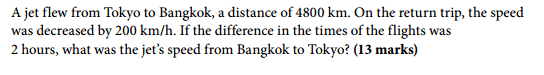none

## The Attempt at a Solution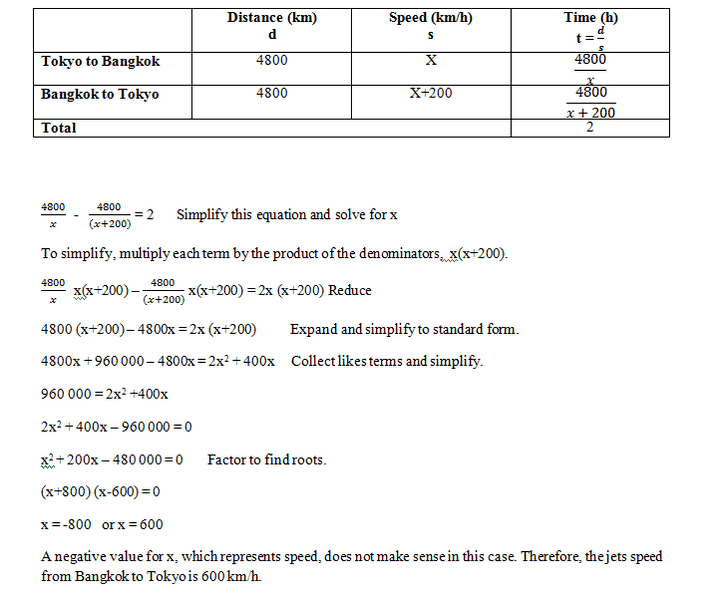Is this correct?

Thanks.

Evangeline101 said:

## Homework Statement

[ ATTACH=full]101053[/ATTACH]

none

## The Attempt at a Solution

[ ATTACH=full]101054[/ATTACH]

Is this correct?

Thanks.

There are errors in your work starting with the table.

If x is the speed from Tokyo to Bangkok then reducing that by 200 gives a speed of x - 200 from Bangkok to Tokyo. Etc.

Evangeline101 said:

## Homework Statement

View attachment 101053

none

## The Attempt at a Solution

View attachment 101054

Is this correct?

Thanks.

Plug it in and see if it works.

SammyS said:

There are errors in your work starting with the table.

If x is the speed from Tokyo to Bangkok then reducing that by 200 gives a speed of x - 200 from Bangkok to Tokyo. Etc.

I understand where I made the error, the return trip speed decreased by 200 km/h, so it should have been (x-200) not (x+200), so I have corrected my answer: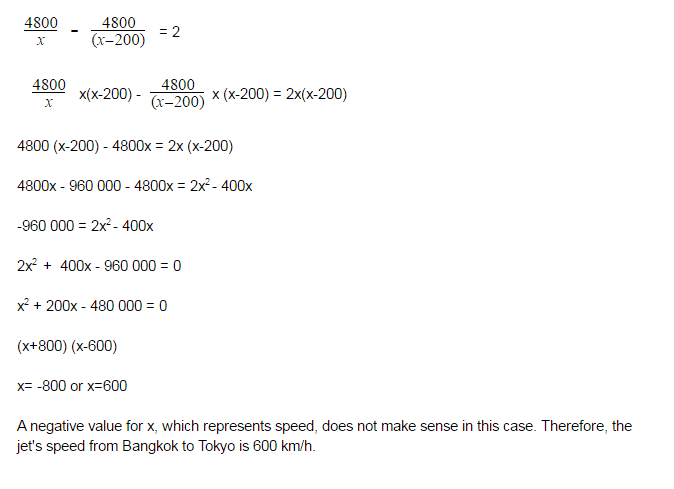Thanks for the help! :)

Evangeline101 said:
I understand where I made the error, the return trip speed decreased by 200 km/h, so it should have been (x-200) not (x+200), so I have corrected my answer:

[ ATTACH=full]101104[/ATTACH]

Thanks for the help! :)
There's still a problem, x is the Tokyo to Bangkok speed. That can't be 600mph. 600 - 200 is 400, and that doesn't work out.

So, where is the problem?

You made a couple of errors.

Sign errors in going from
##-960000=2x^2-400x ##​
to
##2x^2+400x-960000= 0 ##​
It should be
##2x^2-400x+960000= 0 ##​
But there is no real solution to that.

The problem is that it's the Tokyo to Bangkok time that's smaller, because the speed is greater. When you subtract to get 2 hours, you need to subtract in the opposite order.

SammyS said:
There's still a problem, x is the Tokyo to Bangkok speed. That can't be 600mph. 600 - 200 is 400, and that doesn't work out.

So, where is the problem?

You made a couple of errors.

Sign errors in going from
−960000=2x2−400x−960000=2x2−400x-960000=2x^2-400x to
2x2+400x−960000=02x2+400x−960000=02x^2+400x-960000= 0 It should be
2x2−400x+960000=02x2−400x+960000=02x^2-400x+960000= 0 But there is no real solution to that.

I tried doing it this way, but as you said there is no real solution.

SammyS said:
The problem is that it's the Tokyo to Bangkok time that's smaller, because the speed is greater. When you subtract to get 2 hours, you need to subtract in the opposite order.

I don't understand what you mean, can you please explain?

Evangeline101 said:
I tried doing it this way, but as you said there is no real solution.

I don't understand what you mean, can you please explain?
If x > 200, then
##\displaystyle \frac{4800}{x-200}>\frac{4800}{x}##​
.

SammyS said:
If x > 200, then
4800x−200>4800x4800x−200>4800x\displaystyle \frac{4800}{x-200}>\frac{4800}{x}.

So even if I switch these two, can I not get the same answer I had originally? How does switching the two help with the answer to the problem? or is it just the proper way to write it?

Evangeline101 said:
So even if I switch these two, can I not get the same answer I had originally? How does switching the two help with the answer to the problem? or is it just the proper way to write it?
It's what is needed of you want to make everything consistent.

As I stated in my first reply, the answer you gave for the time from Bangkok to Tokyo was correct. (600 km/hr)

But you had a number of inconsistencies.

You should get x = 800. Then x- 200 = 600, the answer.

Last edited:
SammyS said:
It's what is needed of you want to make everything consistent.

As I stated in my first reply, the answer you gave for the time from Bangkok to Tokyo was correct. (600 km/hr)

But you had a number of inconsistencies.

You should get x = 800. Then x- 200 = 600, the answer.

So like this: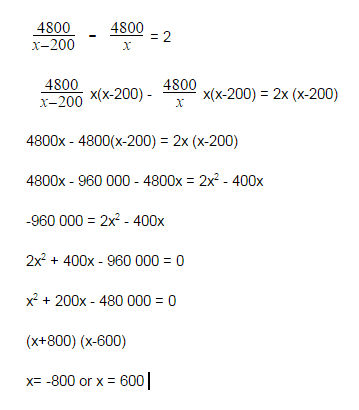Evangeline101 said:
So like this:

View attachment 101169
No.Not like that.
The first three lines are correct.

After that, there are at least two Algebra mistakes.

As I stated in my previous post:
SammyS said:
You should get x = 800. Then x- 200 = 600, the answer.

This is an example from my lesson that I have been using as a reference to solve my problem: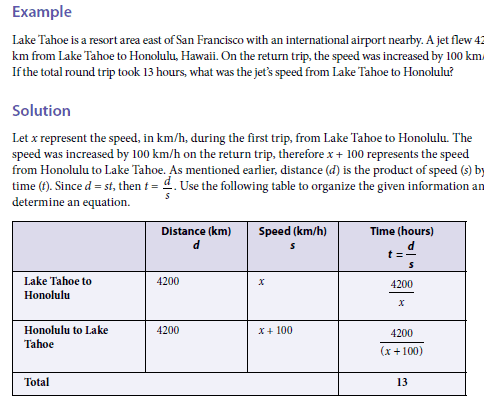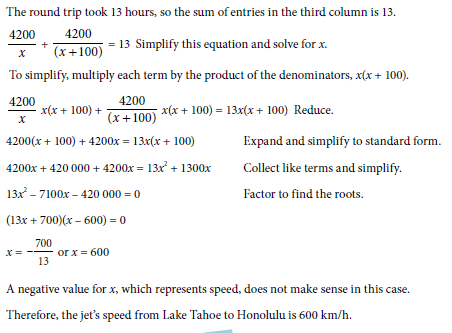Evangeline101 said:
This is an example from my lesson that I have been using as a reference to solve my problem:
View attachment 101173

View attachment 101174
Right. And they did not make algebra mistakes. You did.

So (carefully) redo your algebra, starting at the third line of the following (from post #10), which is the last line that's correct.SammyS said:
No.Not like that.
The first three lines are correct.

After that, there are at least two Algebra mistakes.4800x - 4800x + 960 000 = 2x2 - 400x (960 000 is positive because I multiplied -4800 and -200)

960 000 = 2x2 - 400x (Now I move 960 000 to the right side and it becomes -960 000)

2x2 - 400x - 960 000 = 0

x2 - 200x - 480 000 = 0

(x+600) (x-800)

x= -600 or x = 800

Does this make more sense? did I fix the algebra errors? if not please show me where I made errors.

#### Attachments

Evangeline101 said:
View attachment 101177

4800x - 4800x + 960 000 = 2x2 - 400x (960 000 is positive because I multiplied -4800 and -200)

960 000 = 2x2 - 400x (Now I move 960 000 to the right side and it becomes -960 000)

2x2 - 400x - 960 000 = 0

x2 - 200x - 480 000 = 0

(x+600) (x-800) = 0

x= -600 or x = 800

Does this make more sense? did I fix the algebra errors? if not please show me where I made errors.
Yes. That looks to be correct.

Okay, so does that make the speed from Bangkok to Tokyo 800 km/h instead of 600 km/h since it is positive?

SammyS said:
You should get x = 800. Then x- 200 = 600, the answer.

So since x = 800, we need to solve for the jets's speed from Bangkok to Tokyo, so (like you said) sub x = 800 into x- 200, which gives:
800 - 200 = 600 km/h

So the jet's speed from Bangkok to Tokyo is 600 km/h.

•SammyS
Thanks for the help, I really appreciate it :)